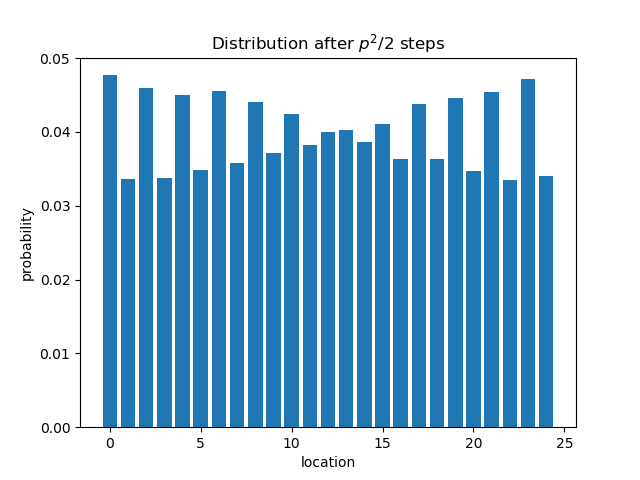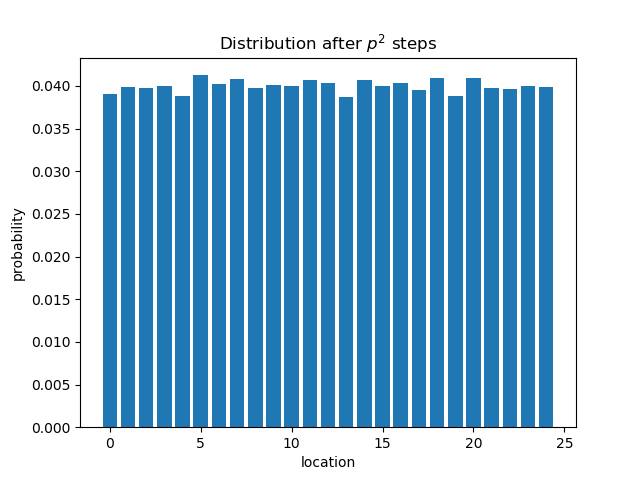# Convergence rate of random walk on integers mod p

Consider a random walk on the integers mod p. At each step, you either take a step forward or a step back, with equal probability. After just a few steps, you’ll have to be near where you started. After a few more steps, you could be far from your starting point, but you’re probably not. But eventually, every point is essentially equally likely.

How many steps would you have to take before your location is uniformly distributed? There’s a theorem that says you need about p² steps. We’ll give the precise statement of the theorem shortly, but first let’s do a simulation.

We’ll take random walks on the integers mod 25. You could think of this as a walk on a 24-hour clock, with one extra hour squeezed in. Suppose we want to take N steps. We would generate a +1 or -1 at random, add that to our current position, and reduce mod 25, doing that N times. That would give us one outcome of a random walk with N steps. We could do this over and over, keeping track of where each random walk ended up, to get an idea how the end of the walk is distributed.

We will do an optimization to speed up the simulation. Suppose we generated all our +1s and -1s at once. Then we just need to add up the number of +1’s and subtract the number of -1’s. The number n of +1’s is a binomial(N, 1/2) random variable, and the number of +1’s minus the number of -1’s is 2nN. So our final position will be

(2nN) mod 25.

Here’s our simulation code.

```    from numpy import zeros
from numpy.random import binomial
import matplotlib.pyplot as plt

p = 25
N = p*p//2
reps = 100000

count = zeros(p)

for _ in range(reps):
n = 2*binomial(N, 0.5) - N
count[n%p] += 1

plt.bar(range(p), count/reps)
plt.show()
```

After doing half of p² steps the distribution is definitely not uniform.But after p² steps the distribution is close to uniform, close enough that you start to wonder how much of the lack of uniformity is due to simulation.Now here’s the theorem I promised, taken from Group Representations in Probability and Statistics by Persi Diaconis.

For np² with p odd and greater than 7, the maximum difference between the random walk density after n steps and the uniform density is no greater than exp(- π²n / 2p²).

There is no magic point at which the distribution suddenly becomes uniform. On the other hand, the difference between the random walk density and the uniform density does drop sharply at around p² steps. Between p²/2 steps and p² steps the difference drops by almost a factor of 12.

## One thought on “Convergence rate of random walk on integers mod p”

1. Jonathan

That Diaconis result initally looks like one of those things that you’d expect: exponential decay with p acting as a scaling factor. But looking at it again I’m very surprised to see a pi^2 inside the exponential. I’m used to seeing pi in front as a normalization factor, that’s unusual for me. I’m almost curious enough to try to track it down, although not “by a \$140 paperback from Amazon” curious.